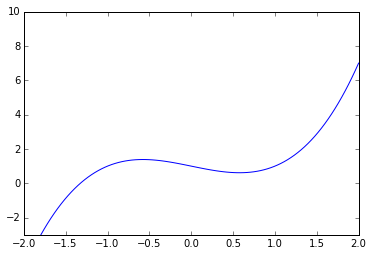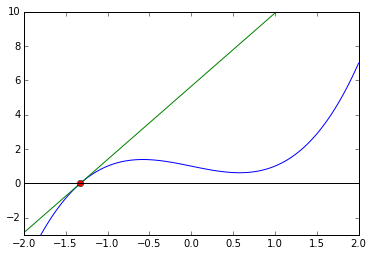# Newton's Method¶

In :
import numpy as np
import matplotlib.pyplot as pt


Here's a function:

In :
def f(x):
return x**3 - x +1

def df(x):
return 3*x**2 - 1

xmesh = np.linspace(-2, 2, 100)
pt.ylim([-3, 10])
pt.plot(xmesh, f(xmesh))

Out:
[<matplotlib.lines.Line2D at 0x7f981263d630>]In :
guesses = 


Evaluate this cell many times in-place (using Ctrl-Enter)

In :
x = guesses[-1] # grab last guess

slope = df(x)

# plot approximate function
pt.plot(xmesh, f(xmesh))
pt.plot(xmesh, f(x) + slope*(xmesh-x))
pt.plot(x, f(x), "o")
pt.ylim([-3, 10])
pt.axhline(0, color="black")

# Compute approximate root
xnew = x - f(x) / slope
guesses.append(xnew)
print(xnew)

-1.324717957244746